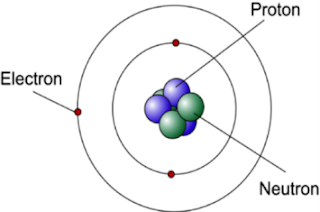# Atomic Structure, Electron Configuration, Valence and free Electron

According to the modern theory, matter is electrical in nature. All the materials are composed of very small particles called atoms.

## Atomic structure

According to the modern theory, matter is electrical in nature. All the materials are composed of very small particles called atoms. The atoms are the building bricks of all matter. An atom consists of a central nucleus of a positive charge around which small negative charged particles, called electrons revolve in different paths or orbits.Structure of Atom

### (i) Nucleus

It is the central part of an atom and contains protons and neutrons. A proton is a positively charged particle, while the neutron has the same mass as the proton, but has no charge. Therefore, the nucleus of an atom is positively charged. The sum of protons and neutrons constitutes the entire weight of an atom and is called atomic weight.

Atomic weight = No. of protons + No. of neutrons

A = p + n

### (ii) Orbit

It is the outer part of an atom and contains electrons only. An electron is a negatively charged particle having negligible mass. The charge on an electron is equal but opposite to that on a proton. Also, the number of electrons is equal to the number of protons in an atom under ordinary conditions. The number of protons or electrons in an atom is called atomic number i.e.

Atomic number = No. of protons or electrons in an atom

Z = p = e

The electrons in an atom revolve around the nucleus in different orbits or paths. The number and arrangement of electrons in any orbit is determined by the following rules:

(a) The number of electrons in any orbit is given by 2n2where n is the number of the orbit. For example,

First orbit contains 2 x 12 = 2 election

Second orbit contains 2 x 22 = 8 elections

Third orbit contains 2 x 32 = 18 electrons

and so on....

(b) The last orbit cannot have more than 8 elections.

(c) The last but one orbit cannot have more than 18 electrons.

## Structure of elements

We have seen that all atoms are made up of protons, neutrons and electrons. The difference between various types of elements is due to the different number and arrangement of these particles within their atoms.Structure of Copper

The atomic structure can be easily build up if we know the atomic weight and atomic number of the element. Thus taking the case copper atom,

Atomic weight = 64

Atomic number = 29

Thus, Number of protons = No. of electrons = 29

and No. of neutrons = 64-29 = 35

Some of the important properties of an electron are :

1. Charge on an electron, e = 1.6×10-19 C
2. Mass of an electron, me = 9.1×10-31 kg
3. Charge on a proton, p = 1.6×10-19 C
4. Mass of a proton, mp = 1.67×10-27 kg
5. Charge on a neutron, n = 0 C
6. Mass of a neutron, mn = 1.67×10-27 kg

## Valence Electrons

The outermost orbit of an atom are known as valence electrons. The outermost orbit can have a maximum of 8 electrons i.e. the maximum number of valence electrons can be 8. The valence electrons determine the physical and chemical properties of a material. These electrons determine whether or not the material is chemical active; metal or non-metal or, a gas or solid. These electrons also determine the electrical properties of a material.

## Free Electrons

The balance electrons which are very loosely attached to the nucleus are known as free electrons. The free electrons can be easily removed or detached by applying a small amount of external energy. As a matter of fact, these are the free electrons which determine the electrical conductivity of a material. On this basis, conductors, insulators and semiconductors can be defined as under :

(i) A conductor is a substance which has a large number of free electrons. When potential difference is applied across a conductor, the free electrons move towards the positive terminal of supply, constituting electric current.

(ii) An insulator is a substance which has practically no fee electrons at ordinary temperature. Therefore, an insulator does not conduct current under the influence of potential difference.

(iii) A semiconductor is a substance which has very few free electrons at room temperature. Consequently, under the influence of potential difference, a semiconductor practically conducts no current.